## Mechanical Advantage, Velocity Ratio and Efficiency

Subject: Science

#### Overview

Mechanical advantage is defined as the ratio of resistance overcomes to the effort applied. Velocity ratio of simple machine is the ratio of distance travelled by the effort to the distance travelled by load in a machine. The efficiency of a simple machine is defined as the ratio of useful work done by machine (output work) to the total work put into the machine (input work). This note provides us with an information about mechanical advantage, velocity ratio and efficiency.
##### Mechanical Advantage, Velocity Ratio and Efficiency

Mechanical advantage is defined as the ratio of resistance overcomes to the effort applied. The simple machine requires force to do work. The resistive force to be overcome is called load and the force applied to overcome the load is called effort.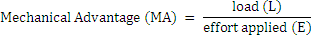#### Velocity RatioVelocity ratio of simple machine is the ratio of distance travelled by the effort to the distance travelled by theload in the machine. As velocity ratio or ideal mechanical advantage is a simple ratio of two distances, it also does not have the unit. The friction is not involved in it.

If a machine overcomes a load ‘L’ and the distance travelled by the load is ‘Ld’. Similarly, the effort applied in the machine is ‘E’ and the distance travelled by effort is ‘Ed’, and 'T' is the time taken then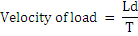#### EfficiencyIf a machine overcomes a load ‘L’ and the distance travelled by the load is ‘Ld’, the work done by the load is L × Ld. It is also called output work or useful work. Therefore,

Output work = L × Ld

Likewise, the effort applied to overcome the load is E and the distance covered by effort is Ed, the work done by effort is E × Ed. It is also called input work. Therefore,

Input work = E × Ed

The efficiency of a simple machine is defined as the ratio of useful work done by a machine (output work) to the total work put into the machine (input work).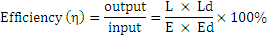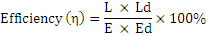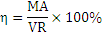For ideal or perfect machine, work output is equal to the work input. Ideal machines are those imaginary machines which are frictionless. In practice, the work output of a machine is always less than work input due to the effect of friction. If the frictional force in the machine increases the efficiency decreases because machines are frictionless in practice, the efficiency of a machine can never be 100%.

##### Things to remember
• Mechanical advantage is defined as the ratio of resistance overcomes to the effort applied.
• Velocity ratio of simple machine is the ratio of distance travelled by effort to the distance travelled by load in the machine.
• The efficiency of a simple machine is defined as the ratio of useful work done by machine (output work) to the total work put into the machine (input work).
• For ideal or perfect machine, work output is equal to the work input but  in practice, the work output of a machine is always less than work input due to the effect of friction.
• It includes every relationship which established among the people.
• There can be more than one community in a society. Community smaller than society.
• It is a network of social relationships which cannot see or touched.
• common interests and common objectives are not necessary for society.
##### Videos for Mechanical Advantage, Velocity Ratio and Efficiency

Mechanical advantage is defined as the ratio of resistance overcome to the effort applied. Simple machine requires force to do work.Velocity ratio of simple machine is defined as the ratio of distance travelled by effort to the distance travelled by load in the machine.

####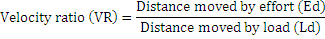The efficiency of a simple machine is defined as the ratio of useful work done by machine (output work) to the total work put into machine (input work).Ideal machine is defined as frictionless machine in which work output is equal to work input and its efficiency is 100%. There is no ideal machine till date as efficiency is never 100%.

If a machine overcomes a load ‘L’ and the distance travelled by the load is ‘Ld’, the work done by the load is L × Ld. It is also called output work

The effort applied to overcome the load is E and the distance covered by effort is Ed, the work done by effort is E × Ed. It is also called input work.

Solution,

\begin{align*} \text {Effort} \: (E)=50N \\ \text {Mechanical advantage}(MA)=4\\\text {Load}\:(L)=? \\ \text {According to formula,} \\ \text {mecchanical advantage}\:(M.A)\: &=\frac {\text {load}}{\text {effort}}\\ \text {load} &= \frac {\text {mechanical advantage}}{\text {effort}} \\ &= 50\: N \times 4 \\ &= 200 N \\ \end{align*}

\begin{align*} \text {load} (l) = 100\: N \\ \text {effort} (E) = 50\: N \\ \text {load distance} (Ld) =10\: m\\ \text {Effort distance} (Ed) = 5\:m \\ \text {we know} \\ \text {mechanical advantage} =\frac {\text {load}}{\text {effort}} = \frac {100}{50} = 2 \\ \text {velocity ratio} &= \frac{\text {load distance}}{\text {effort distance}} = \frac {10\:m}{2\: m} = 5 \\ \text {efficiency} &= \frac {\text {mechanical advantage}}{\text {velocity ratio}} \times 100\% \\ &= \frac 25 \times 100\% \\ \text {efficiency} &= 40\% \\ \end{align*}